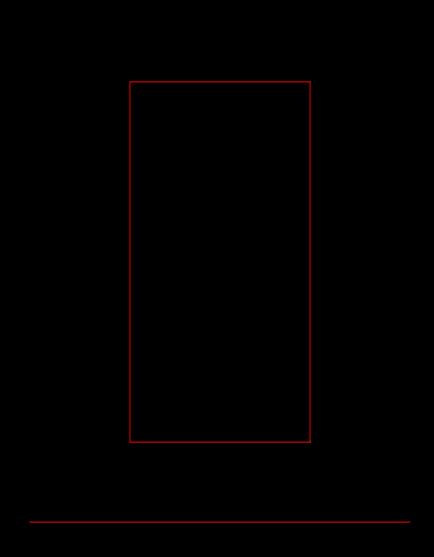## Description

This tool is used to generate either a quad/rectangle or a line. For this purpose, two points are used to define the geometry. To create a quad, the defining points need to be in a plane parallel to the XY-, YZ- or YZ-plane. To create a line, they must define a line parallel to the standard basis vectors of 3D space. Quads and lines then can be combined to create more complex geometries. This tool is mainly used to create simple geometries for benchmarking or testing purposes.

## Usage

USAGE:
generateGeometry  -o <output file>
[--polyline_name <name of the generated polyline>]
[--geometry_name <name of the geometry>]
[--nz1 <number of subdivisions in z direction>]
[--nz <number of subdivisions in z direction>]
[--ny1 <number of subdivisions in y direction>]
[--ny <number of subdivisions in y direction>]
[--nx1 <number of subdivisions in x direction>]
[--nx <number of subdivisions in x direction>]
--x1 <x1> --y1 <y1> --z1 <z1> --x0 <x0> --y0 <y0> --z0 <z0>
[--] [--version] [-h]

Where:

-o <output file>,  --output <output file>
(required)  output geometry file (*.gml)

--polyline_name <name of the generated polyline>
name of the generated polyline

--geometry_name <name of the geometry>
name of the generated geometry

--nz1 <number of subdivisions in z direction>
number of subdivisions in z direction

--nz <number of subdivisions in z direction>
number of subdivisions in z direction

--ny1 <number of subdivisions in y direction>
number of subdivisions in y direction

--ny <number of subdivisions in y direction>
number of subdivisions in y direction

--nx1 <number of subdivisions in x direction>
number of subdivisions in x direction

--nx <number of subdivisions in x direction>
number of subdivisions in x direction

--x1 <x1>
(required)  x coordinate of the first point

--y1 <y1>
(required)  y coordinate of the first point

--z1 <z1>
(required)  z coordinate of the first point

--x0 <x0>
(required)  x coordinate of the first point

--y0 <y0>
(required)  y coordinate of the first point

--z0 <z0>
(required)  z coordinate of the first point

--,  --ignore_rest
Ignores the rest of the labeled arguments following this flag.

--version
Displays version information and exits.

-h,  --help
Displays usage information and exits.

Subdivisions can be made along all 4 edges of a quad. The input is a number that defines the amount of equidistant points that are created on the corresponding edge/line. When a mesh is generated using this geometry, these points are also integrated into the mesh. Generating subdivisions along a line is done by --nx, --ny or --nz depending on the axis the line is parallel to.

## Example

In this example we generate a line by defining two points p0 = (-4,-2,3) and p1= (15,-2,3). Here, a line is generated because y0 = y1 = -2 and z0 = z1 = 3 for the two points. This means the defined points are in a line parallel to the x-axis.

generateGeometry -o line.gml --x0 -4 --x1 15 --y0 -2 --y1 -2 --z0 3 --z1 3

In this example we generate a quad by defining two points p0 = (1,2,3) and p1= (10,20,3). Here, a plane is generated because z0 = z1 = 3 for the two points. This means the defined points are in a plane parallel to x-y-plane.

generateGeometry -o quad.gml --x0 1 --x1 10 --y0 2 --y1 20 --z0 3 --z1 3Fig.1 Visualization of the generated quad and the line viewed along the z-axis.

This article was written by Julian Heinze. If you are missing something or you find an error please let us know.
Generated with Hugo 0.117.0 in CI job 362666 | Last revision: July 12, 2023
Commit: [PL/SSD] Rename x_dot to x_prev 04a8fec8  | Edit this page on